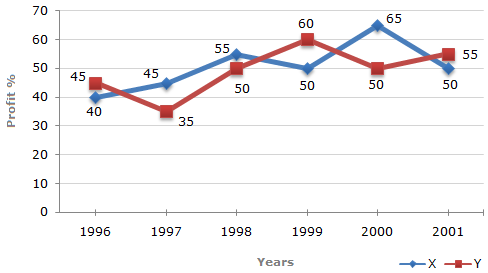# Data Interpretation - Line Charts - Discussion

Discussion Forum : Line Charts - Line Chart 4 (Q.No. 2)
Directions to Solve

The following line graph gives the percent profit earned by two Companies X and Y during the period 1996 - 2001.

Percentage profit earned by Two Companies X and Y over the Given Years

 %Profit = Income - Expenditure x 100 Expenditure2.
If the expenditure of Company Y in 1997 was Rs. 220 crores, what was its income in 1997 ?
Rs. 312 crores
Rs. 297 crores
Rs. 283 crores
Rs. 275 crores
Explanation:

Profit percent of Company Y in 1997 = 35.

Let the income of Company Y in 1997 be Rs. x crores.

 Then, 35 = x - 220 x 100x = 297. 220Income of Company Y in 1997 = Rs. 297 crores.

Discussion:
8 comments Page 1 of 1.

Varshini said:   2 years ago
35 = [(x-220)/220*100].
35/100 = (x-220)/220.
(35/100)*220 = x - 220,
0.35*220 = x-220,
77 = x-220,
x = 220 + 77,
x = 297.
(4)

Raju said:   5 years ago
Anyone, please explain the given chart.

Sara said:   6 years ago
@Jija.

It's given in the chart. Please check it.

Jija said:   6 years ago
How 35 comes?

Sandeep said:   8 years ago
220 of 135% = 297.
(1)

Swathi said:   9 years ago
35 = x-220/220*100.
x-220/220 = 35/100.
x-220/220 = 7/20.
20x-4400 = 1540.
20x = 1540+4400.
20x = 5940.
x = 5940/20.
x = 297.

Kumar said:   9 years ago
See here,
35 = (x/220-220/220)*100.

35 = (x/220-1)*100.
35/100 = x/220 -1.

35/100+1 = x/220.
135/100 = x/220.

135100*220 = x.
297 = x.

Ramrsh said:   10 years ago